# Refractive index

Refractive index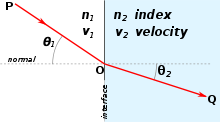Refraction of light at the interface between two media.

In optics the refractive index or index of refraction of a substance or medium is a measure of the speed of light in that medium. It is expressed as a ratio of the speed of light in vacuum relative to that in the considered medium.   This can be written mathematically as:

n = speed of light in a vacuum / speed of light in medium.

For example, the refractive index of water is 1.33, meaning that light travels 1.33 times as fast in vacuum as it does in water. (See typical values for different materials here.)

As light moves from a medium, such as air, water, or glass, into another it may change its propagation direction in proportion to the change in refractive index. This refraction is governed by Snell's law, and is illustrated in the figure to the right. Refractive index of materials varies with the wavelength of light. This is called dispersion and results in a slightly different refractive index for each color.

The wavelength λ of light in a material is determined by the refractive index according to λ = λ0 / n, where λ0 is the wavelength of the light in vacuum. Brewster's angle, the critical angle for total internal reflection, and the reflectivity of a surface is also affected by the refractive index. These material parameters can be calculated using the Fresnel equations.

The concept of refractive index is widely used within the full electromagnetic spectrum, from x-rays to radio waves. It can also be used with wave phenomena other than light, e.g. sound. In this case the speed of sound is used instead of that of light and a reference medium other than vacuum must be chosen.

## Definitions

The refractive index, n, of a medium is defined as the ratio of the speed, c, of a wave phenomenon such as light or sound in a reference medium to the phase speed, vp, of the wave in the medium in question:$n = \frac{c}{v_{\mathrm {p}}}.$

It is most commonly used in the context of light with vacuum as a reference medium, although historically other reference media (e.g. air at a standardized pressure and temperature) have been common. It is usually given the symbol n. In the case of light, it equals$n=\sqrt{\epsilon_r\mu_r},$

where$\epsilon_r\;$ is the material's relative permittivity, and μr is its relative permeability. For most naturally occurring materials, μr is very close to 1 at optical frequencies, therefore n is approximately$\sqrt{\epsilon_r}$. Contrary to a widespread misconception, the real part of a complex n may be less than one, depending upon the material and wavelength (see dispersion (optics)). This has practical technical applications, such as effective mirrors for X-rays based on total external reflection.

The phase speed is defined as the rate at which the crests of the waveform propagate; that is, the rate at which the phase of the waveform is moving. The group speed is the rate at which the envelope of the waveform is propagating; that is, the rate of variation of the amplitude of the waveform. Provided the waveform is not distorted significantly during propagation, it is the group speed that represents the rate at which information (and energy) may be transmitted by the wave (for example, the speed at which a pulse of light travels down an optical fiber). For the analytic properties constraining the unequal phase and group speeds in dispersive media, refer to dispersion (optics).

Another common definition of the refractive index comes from the refraction of a light ray entering a medium. The refractive index is the ratio of the sines of the angles of incidence θ1 and refraction θ2 as light passes into the medium or mathematically$n=\frac{\sin\theta_1}{\sin\theta_2}.$

The angles are measured to the normal of the surface. This definition is based on Snell's law and is equivalent to the definition above if the light enters from the reference medium (normally vacuum).

A complex refractive index is often used to take absorption into account. This is further discussed in the Dispersion and absorption section below.

A closely related quantity is refractivity, which in atmospheric applications is denoted N and defined as N = 106(n - 1). The 106 factor is used because for air, n deviates from unity at most a few parts per thousand.

## Typical values

Selected refractive indices at λ = 589nm (more extensive list here).
Material n
Gases at 0 °C and 1 atm
Air 1.000293
Helium 1.000036
Hydrogen 1.000132
Carbon dioxide 1.00045
Liquids at 20 °C
Water 1.333
Ethanol 1.36
Methanol 1.329
Benzene 1.501
Glycerine 1.473
Solids
Ice 1.309
Fused silica 1.46
PMMA 1.49
Crown glass (typical) 1.52
Flint glass (typical) 1.62
Diamond 2.42

For visible light most transparent media have refractive indices between 1 and 2. A few examples are given in the table to the right. These values are measured at the yellow doublet sodium D-line, with a wavelength of 589 nanometres, as is conventionally done. For infrared light refractive indices can be considerably higher. Germanium is transparent in this region and has a refractive index of about 4, making it an important material for infrared optics.

### Refractive index below 1

A widespread misconception is that since, according to the theory of relativity, nothing can travel faster than the speed of light in vacuum, the refractive index cannot be lower than 1. This is erroneous since the refractive index measures the phase velocity of light, which does not carry energy or information, the two things limited in propagation speed. The phase velocity is the speed at which the crests of the wave move and can be faster than the speed of light in vacuum, and thereby give a refractive index below 1. This can occur close to resonance frequencies, in plasmas, and for x-rays. In the x-ray regime the refractive indices are lower than but very close to 1 (exceptions close to some resonance frequencies). As an example, water has a refractive index of 1−2.6×10−7 at a photon energy of 30 keV (0.04 nm wavelength).

### Negative refractive index

Recent research has also demonstrated the existence of the negative refractive index, which can occur if permittivity and permeability have simultaneous negative values. This can be achieved with periodically constructed metamaterials. The resulting negative refraction (i.e., a reversal of Snell's law) offers the possibility of the superlens and other exotic phenomena.

## Microscopic explanation

At the microscale, an electromagnetic wave's phase speed is slowed in a material because the electric field creates a disturbance in the charges of each atom (primarily the electrons) proportional to the electric susceptibility of the medium. (Similarly, the magnetic field creates a disturbance proportional to the magnetic susceptibility.) As the electromagnetic fields oscillate in the wave, the charges in the material will be "shaken" back and forth at the same frequency. The charges thus radiate their own electromagnetic wave that is at the same frequency, but usually with a phase delay, as the charges may move out of phase with the force driving them (see sinusoidally-driven harmonic oscillator). The light wave traveling in the medium is the macroscopic superposition (sum) of all such contributions in the material: The original wave plus the waves radiated by all the moving charges. This wave is typically a wave with the same frequency but shorter wavelength than the original, leading to a slowing of the wave's phase speed. Most of the radiation from oscillating material charges will modify the incoming wave, changing its velocity. However, some net energy will be radiated in other directions or even at other frequencies (see scattering).

Depending on the relative phase of the original driving wave and the waves radiated by the charge motion, there are several possibilities:

• If the electrons emit a light wave which is 90° out of phase with the light wave shaking them, it will cause the total light wave to travel more slowly. This is the normal refraction of transparent materials like glass or water, and corresponds to a refractive index which is real and greater than 1.
• If the electrons emit a light wave which is 270° out of phase with the light wave shaking them, it will cause the total light wave to travel more quickly. This is called "anomalous refraction", and is observed close to absorption lines, with X-rays, and in some microwave systems. It corresponds to a refractive index less than 1. (Even though the phase velocity of light is greater than the speed of light in vacuum c, the signal velocity is not, as discussed above). If the response is sufficiently strong and out-of-phase, the result is negative refractive index discussed below.
• If the electrons emit a light wave which is 180° out of phase with the light wave shaking them, it will destructively interfere with the original light to reduce the total light intensity. This is light absorption in opaque materials and corresponds to an imaginary refractive index.
• If the electrons emit a light wave which is in phase with the light wave shaking them, it will amplify the light wave. This is rare, but occurs in lasers due to stimulated emission. It corresponds to an imaginary index of refraction, with the opposite sign as absorption.

For most materials at visible-light frequencies, the phase is somewhere between 90° and 180°, corresponding to a combination of both refraction and absorption.

## Dispersion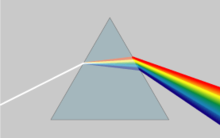In a prism dispersion causes different colors to refract at different angles, splitting white light into a rainbow of colors.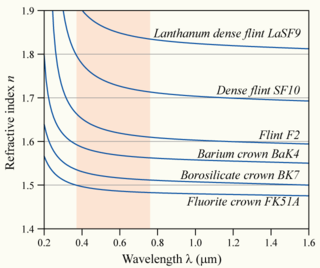The variation of refractive index with wavelength for various glasses.

The refractive index of materials varies with the wavelength (and frequency) of light. This is called dispersion and causes prisms to divide white light into its constituent spectral colors, and explains how rainbows are formed. As the refractive index varies with wavelength, according to Snell's law, so will the refraction angle as light goes from one material to another. This makes different colors go in different directions. Dispersion also causes the focal length of lenses to be wavelength dependent. This is a type of chromatic aberration, which often needs to be corrected for in imaging systems.

In regions of the spectrum where the material does not absorb, the refractive index tends to decrease with increasing wavelength, and thus increase with frequency. This is called normal dispersion, in contrast to anomalous dispersion, where the refractive index increases with wavelength. For visible light normal dispersion means that the refractive index is higher for blue light than for red.

For optics in the visual range the amount of dispersion of a lens material is often quantified by the Abbe number$V=\frac{n_\text{yellow}-1}{n_\text{blue}-n_\text{red}}$. For a more accurate description of the wavelength dependence of the refractive index the Sellmeier equation can be used. It is an empirical formula that works well in describing dispersion. Sellmeier coefficients are often quoted instead of the refractive index in tables.

Because of dispersion, it is usually important to specify the vacuum wavelength at which a refractive index is measured. Typically, this is done at various well-defined spectral emission lines; for example, nD is the refractive index at the Fraunhofer "D" line, the centre of the yellow sodium double emission at 589.29 nm wavelength.

## Complex index of refraction and absorption

When light passes through a medium, some part of it will always be absorbed. This can be conveniently taken into account by defining a complex index of refraction,$\tilde{n}=n+i\kappa.$

Here, the real part of the refractive index n indicates the phase speed, while the imaginary part κ indicates the amount of absorption loss when the electromagnetic wave propagates through the material.

That κ corresponds to absorption can be seen by inserting this refractive index into the expression for electric field of a plane electromagnetic wave traveling in the z-direction. We can do this by relating the wave number to the refractive index through$k = \tfrac{2\pi n}{\lambda_0}$, with λ0 being the vacuum wavelength. With complex wave number$\tilde{k}$ and refractive index n + iκ this can be inserted into the plane wave expression as$\mathbf{E}(z,t) = \mathrm{Re} (\mathbf{E}_0 e^{i(\tilde{k} z - \omega t)}) = \mathrm{Re} (\mathbf{E}_0 e^{i(2\pi(n+i\kappa)z/\lambda_0 - \omega t)}) = e^{-2\pi\kappa z/\lambda_0} \mathrm{Re} (\mathbf{E}_0 e^{i(kz - \omega t)}).$

Here we see that κ gives an exponential decay, as expected from the Beer–Lambert law.

κ is often called the extinction coefficient in physics although this has a different definition within chemistry. Both n and κ are dependent on the frequency. In most circumstances κ > 0 (light is absorbed) or κ = 0 (light travels forever without loss). In special situations, especially in the gain medium of lasers, it is also possible that κ < 0, corresponding to an amplification of the light.

An alternative convention uses$\tilde{n}=n-i\kappa$ instead of$\tilde{n}=n+i\kappa$, but where κ > 0 still corresponds to loss. Therefore these two conventions are inconsistent and should not be confused. The difference is related to defining sinusoidal time dependence as Re(e iωt) versus Re(e + iωt). See Mathematical descriptions of opacity.

Dielectric loss and non-zero DC conductivity in materials cause absorption. Good dielectric materials such as glass have extremely low DC conductivity, and at low frequencies the dielectric loss is also negligible, resulting in almost no absorption (κ ≈ 0). However, at higher frequencies (such as visible light), dielectric loss may increase absorption significantly, reducing the material's transparency to these frequencies.

The real and imaginary parts of the complex refractive index are related through the Kramers–Kronig relations. For example, one can determine a material's full complex refractive index as a function of wavelength from an absorption spectrum of the material.

For X-ray and extreme ultraviolet radiation the complex refractive index deviates only slightly from unity and usually has a real part smaller than 1. It is therefore normally written as$\tilde{n}=1-\delta+i\beta$ (or$\tilde{n}=1-\delta-i\beta$).

## Relations to other quantities

### RefractionRefraction of light at the interface between two media of different refractive indices, with n2 > n1. Since the phase velocity is lower in the second medium (v2 < v1), the angle of refraction θ2 is less than the angle of incidence θ1; that is, the ray in the higher-index medium is closer to the normal.

When light moves from one medium to another as in the figure to the right, it changes direction, i.e. it is refracted. If it goes from a medium with refractive index n1 to one with refractive index n2, with an incidence angle to the surface normal of θ1, the transmission angle θ2 can be calculated from Snell's law:

n1sin θ1 = n2sin θ2.

If there is no angle θ2 fulfilling Snell's law, i.e.$\frac{n_1}{n_2}\sin\theta_1>1$,

the light cannot be transmitted and will instead undergo total internal reflection.

### Reflectivity

Apart from the transmitted light there is also a reflected part. The reflection angle is equal to the incidence angle, and the amount of light that is reflected is determined by the reflectivity of the surface. The reflectivity can be calculated from the refractive index and the incidence angle with the Fresnel equations, which for normal incidence reduces to$R = \left(\frac{n_1-n_2}{n_1+n_2}\right)^2$.

For common glass in air, n1 = 1 and n2 = 1.5, and thus about 4% of the incident power is reflected. At other incidence angles the reflectivity will also depend on the polarization of the incoming light. At a certain angle called Brewster's angle, p-polarized light (light with the electric field in the plane of incidence) will be totally transmitted. Brewster's angle can be calculated from the two refractive indices of the interface as$\theta_\mathrm B = \arctan \left( \frac{n_2}{n_1} \right).$

### Lenses

The focal length of a lens is determined by its refractive index n and the radii of curvature R1 and R2 of its surfaces. The power of a thin lens in air is given by the Lensmaker's formula:$\frac{1}{f} = \left(n-1\right)\left( \frac{1}{R_1} - \frac{1}{R_2} \right).$

### Dielectric constant

The frequency dependent dielectric constant is simply the square of the (complex) refractive index in a non-magnetic medium (one with a relative permeability of unity). The refractive index is used for optics in Fresnel equations and Snell's law; while the dielectric constant is used in Maxwell's equations and electronics.

Where$\tilde\epsilon$ is the complex dielectric constant with real and imaginary parts$\ \epsilon_1$ and$\ \epsilon_2$, and n and$\ \kappa$ are the real and imaginary parts of the refractive index, all functions of frequency:$\tilde\epsilon=\epsilon_1+i\epsilon_2= (n+i\kappa)^2.$

Conversion between refractive index and dielectric constant is done by:$\ \epsilon_1= n^2 - \kappa^2$$\ \epsilon_2 = 2n\kappa$$\ n = \sqrt{\frac{\sqrt{\epsilon_1^2+\epsilon_2^2}+\epsilon_1}{2}}$$\kappa = \sqrt{ \frac{ \sqrt{ \epsilon_1^2+ \epsilon_2^2}- \epsilon_1}{2}}.$

### Density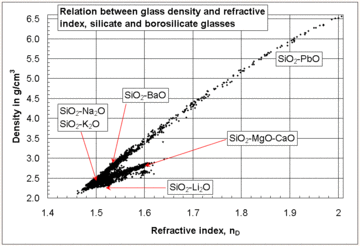Relation between the refractive index and the density of silicate and borosilicate glasses.

In general, the refractive index of a glass increases with its density. However, there does not exist an overall linear relation between the refractive index and the density for all silicate and borosilicate glasses. A relatively high refractive index and low density can be obtained with glasses containing light metal oxides such as Li2O and MgO, while the opposite trend is observed with glasses containing PbO and BaO as seen in the diagram at the right.

### Group index

Sometimes, a "group speed refractive index", usually called the group index is defined:$n_g=\frac{c}{v_g}$

where vg is the group velocity. This value should not be confused with n, which is always defined with respect to the phase velocity. When the dispersion is small, the group velocity can be linked to the phase velocity by the relation$v_g = v - \lambda\frac{dv}{d\lambda}.$

In this case the group index can thus be written in terms of the wavelength dependence of the refractive index as$n_g = \frac{n}{1+\frac{\lambda}{n}\frac{dn}{d\lambda}},$

where λ is the wavelength in the medium.

When the refractive index of a medium is known as a function of the vacuum wavelength (instead of the wavelength in the medium), the corresponding expressions for the group velocity and index are (for all values of dispersion)$v_g = c \left( n - \lambda_0 \frac{dn}{d\lambda_0} \right)^{-1}.$$n_g = n-\lambda_0\frac{dn}{d\lambda_0},$

where λ0 is the wavelength in vacuum.

### Other relations

As shown in the Fizeau experiment, when light is transmitted through a moving medium, its speed relative to a stationary observer is:$V = \frac {c}{n} + v \left(1 - \frac{1}{n^2}\right)$

The refractive index of a substance can be related to its polarizability with the Lorentz–Lorenz equation or to the molar refractivities of its constituents by the Gladstone–Dale relation.

## BirefringenceA calcite crystal laid upon a paper with some letters showing double refraction.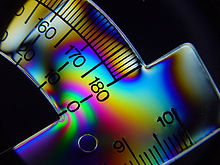Birefringent materials can gives rise to colors when placed between crossed polarizers. This is the basis for photoelasticity.

In some materials the refractive index depends on the polarization and propagation direction of the light. This is called birefringence or optical anisotropy.

In the simplest form, uniaxial birefringence, there is only one special direction in the material. This axis is known as the optical axis of the material. Light with linear polarization perpendicular to this axis will experience an ordinary refractive index no while light polarized in parallel will experience an extraordinary refractive index ne. The birefringence of the material is the difference between these indices of refraction,$\Delta n=n_e-n_o\,$. Light propagating in the direction of the optical axis will not be affected by the birefringence since the refractive index will be no independent of polarization. For other propagation directions the light will split into two linearly polarized beams. For light traveling perpendicularly to the optical axis the beams will have the same direction. This can be used to change the polarization direction of linearly polarized light or to convert between linear, circular and elliptical polarizations with waveplates.

Many crystals are naturally birefringent, but isotropic materials such as plastics and glass can also often be made birefringent by introducing a prefered direction through e.g. an external force or electric field. This can be utilized to modulate light in liquid crystal displays or in the determination of stresses in structures using photoelasticity. In both cases the birefringent material is placed between crossed polarizers. A change in birefringence will alter the polarization and thereby the fraction of light that is transmitted through the second polarizer.

In the more general case of trirefringent materials described by the field of crystal optics, the dielectric constant is a rank-2 tensor (a 3 by 3 matrix). In this case the propagation of light cannot simply be described by refractive indices except for polarizations along principal axes.

## Nonlinearity

The strong electric field of high intensity light (such as output of a laser) may cause a medium's refractive index to vary as the light passes through it, giving rise to nonlinear optics. If the index varies quadratically with the field (linearly with the intensity), it is called the optical Kerr effect and causes phenomena such as self-focusing and self-phase modulation. If the index varies linearly with the field (which is only possible in materials that do not possess inversion symmetry), it is known as the Pockels effect.

## Inhomogeneity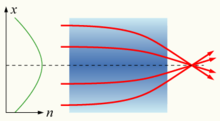A gradient-index lens with a parabolic variation of refractive index (n) with radial distance (x). The lens focuses light in the same way as a conventional lens.

If the refractive index of a medium is not constant, but varies gradually with position, the material is known as a gradient-index medium and is described by gradient index optics. Light traveling through such a medium can be bent or focused, and this effect can be exploited to produce lenses, some optical fibers and other devices. Some common mirages are caused by a spatially-varying refractive index of air.

## Refractive index measurement

### Homogeneous media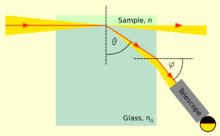The principle of many refractometers.

The refractive index of liquids or solids can be measured with refractometers. They typically measure some angle of refraction or the critical angle for total internal reflection. The first laboratory refractometers sold commercially where developed by Ernst Abbe in the late 19th century. The same principles are still used today. In this instrument a thin layer of the liquid to be measured is placed between two prisms. Light is shone through the liquid at incidence angles all the way up to 90°, i.e. light rays parallel to the surface. The second prism should have an index of refraction higher than that of the liquid, so that light only enters the prism at angles smaller than the critical angle for total reflection. This angle can then be measured either by looking through a telescope, or with a digital photodetector placed in the focal plane of a lens. The refractive index n of the liquid can then be calculated from the maximum transmission angle θ as n = nGsin θ, where nG is the refractive index of the prism.Photo of a handheld refractometer used to measure sugar content of fruits.

This type of devices are commonly used in chemical laboratories for identification of substances and for quality control. Handheld variants are used in agriculture by e.g. wine makers to determine sugar content in grape juice, and inline process refractometers are used in e.g. chemical and pharmaceutical industry for process control.

In gemology a different type of refractometer is used to measure index of refraction and birefringence of gemstones. The gem is placed on a high refractive index prism and illuminated from below. A high refractive index contact liquid is used to achieve optical contact between the gem and the prism. At small incidence angles most of the light will be transmitted into the gem, but at high angles total internal reflection will occur in the prism. The critical angle is normally measured by looking through a telescope.

### Refractive index variations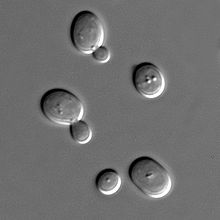A differential interference contrast microscopy image of yeast cells.

To measure the spatial variation of refractive index in a sample phase-contrast imaging methods are used. These methods measure the variations in phase of the light wave exiting the sample. The phase is proportional to the optical path length the light ray has traversed, and thus gives a measure of the integral of the refractive index along the ray path. The phase cannot be measured directly at optical or higher frequencies, and therefore needs to be converted into intensity by interference with a reference beam. In the visual spectrum this is done using Zernike phase-contrast microscopy, differential interference contrast microscopy (DIC) or interferometry.

Zernike phase-contrast microscopy introduces a phase shift to the low spatial frequency components of the image with a phase-shifting annulus in the Fourier plane of the sample, so that higher frequency parts of the image can interfere with the low frequency reference beam. In DIC the illumination is split up into two beams that are given different polarizations, are phase shifted differently, and are shifted transversely with slightly different amounts. After the specimen the two parts are made to interfere giving an image of the derivative of the optical path length in the direction of the difference in transverse shift. In interferometry the illumination is split up into two beams by a partially reflective mirror. One of the beams is let through the sample before they are combined to interfere and give a direct image of the phase shifts. If the optical path length variations are more than a wavelength the image will contain fringes.

There exist several x-ray phase-contrast imaging techniques to determine 2D or 3D spatial distribution of refractive index of samples in the x-ray regime.

## Abraham–Minkowski controversy

In 1908, Hermann Minkowski calculated the momentum of a refracted ray, p, where E is energy of the photon, c is the speed of light in vacuum and n is the refractive index of the medium as follows:$p=\frac{nE}{c} .$

In 1909, Max Abraham proposed the following formula for this calculation:$p=\frac{E}{nc}.$

A 2010 study suggested that both equations are correct, with the Abraham version being the kinetic momentum and the Minkowski version being the canonical momentum, and claims to explain the contradicting experimental results using this interpretation.

## ApplicationsWavefronts from a point source in the context of Snell's law. The region below the gray line has a higher index of refraction, and so light traveling through it has a proportionally lower phase speed than in the region above it.

The refractive index of a material is the most important property of any optical system that uses refraction. It is used to calculate the focusing power of lenses, and the dispersive power of prisms. It can also be used as a useful tool to differentiate between different types of gemstone, due to the unique chatoyance each individual stone displays.

Since refractive index is a fundamental physical property of a substance, it is often used to identify a particular substance, confirm its purity, or measure its concentration. Refractive index is used to measure solids (glasses and gemstones), liquids, and gases. Most commonly it is used to measure the concentration of a solute in an aqueous solution. A refractometer is the instrument used to measure refractive index. For a solution of sugar, the refractive index can be used to determine the sugar content (see Brix).

In GPS, the index of refraction is utilized in ray-tracing to account for the radio propagation delay due to the Earth's electrically neutral atmosphere. It is also used in Satellite link design for the Computation of radiowave attenuation in the atmosphere

Wikimedia Foundation. 2010.

### См. также в других словарях:

• Refractive index — Refractive Re*fract ive (r?*fr?kt ?v), a. [Cf. F. r[ e]fractif. See {Refract}.] Serving or having power to refract, or turn from a direct course; pertaining to refraction; as, refractive surfaces; refractive powers. [1913 Webster] {Refractive… …   The Collaborative International Dictionary of English

• Refractive index — Index In dex, n.; pl. E. {Indexes}, L. {Indices}(?). [L.: cf. F. index. See {Indicate}, {Diction}.] [1913 Webster] 1. That which points out; that which shows, indicates, manifests, or discloses; as, the increasing unemployment rate is an index of …   The Collaborative International Dictionary of English

• refractive index — n INDEX OF REFRACTION * * * the refractive power of a medium compared with that of air, which is assumed to be 1. Symbol n, n, or nD …   Medical dictionary

• refractive index — n. INDEX OF REFRACTION …   English World dictionary

• refractive index — Optics. See index of refraction. [1830 40] * * * ▪ physics also called  Index Of Refraction,         measure of the bending of a ray of light when passing from one medium into another. If i is the angle of incidence of a ray in vacuum (angle… …   Universalium

• refractive index — lūžio rodiklis statusas T sritis automatika atitikmenys: angl. index of refraction; refraction index; refractive index vok. Brechungsindex, m; Brechungsverhältnis, n; Brechungszahl, f; Brechzahl, f; Refraktionsindex, m rus. индекс преломления, m; …   Automatikos terminų žodynas

• refractive index — lūžio rodiklis statusas T sritis chemija apibrėžtis Medžiagos konstanta, apibūdinanti jos savybę laužti šviesos bangas. atitikmenys: angl. index of refraction; refraction index; refractive index rus. индекс преломления; коэффициент рефракции;… …   Chemijos terminų aiškinamasis žodynas

• refractive index — noun the ratio of the velocity of light in a vacuum to that in a medium • Syn: ↑index of refraction • Hypernyms: ↑ratio * * * reˌfractive ˈindex 7 [refractive index] noun …   Useful english dictionary

• refractive index — lūžio rodiklis statusas T sritis Standartizacija ir metrologija apibrėžtis Esant nesugeriančiai terpei, tai elektromagnetinės spinduliuotės sklidimo greičio vakuume ir tam tikro dažnio elektromagnetinės spinduliuotės fazinio greičio terpėje… …   Penkiakalbis aiškinamasis metrologijos terminų žodynas

• refractive index — lūžio rodiklis statusas T sritis Standartizacija ir metrologija apibrėžtis Medžiagos parametras, apibūdinantis jos savybę laužti šviesos bangas. atitikmenys: angl. refraction index; refractive index vok. Brechungsindex, m rus. показатель… …   Penkiakalbis aiškinamasis metrologijos terminų žodynas

### Поделиться ссылкой на выделенное

##### Прямая ссылка:
Нажмите правой клавишей мыши и выберите «Копировать ссылку»